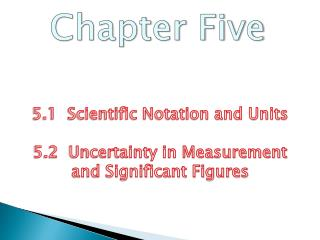Download PresentationChapter Five

Loading in 2 Seconds...

# Chapter Five - PowerPoint PPT Presentation

Chapter Five. 5.1 Scientific Notation and Units 5.2 Uncertainty in Measurement and Significant Figures. 5.1 Objectives. To show how very large or very small numbers can be expressed in scientific notation To learn the English, metric, and SI systems of measurementI am the owner, or an agent authorized to act on behalf of the owner, of the copyrighted work described.
Download Presentation## Chapter Five

An Image/Link below is provided (as is) to download presentation

Download Policy: Content on the Website is provided to you AS IS for your information and personal use and may not be sold / licensed / shared on other websites without getting consent from its author.While downloading, if for some reason you are not able to download a presentation, the publisher may have deleted the file from their server.

- - - - - - - - - - - - - - - - - - - - - - - - - - E N D - - - - - - - - - - - - - - - - - - - - - - - - - -
Presentation Transcript
1. Chapter Five 5.1 Scientific Notation and Units 5.2 Uncertainty in Measurement and Significant Figures

2. 5.1 Objectives • To show how very large or very small numbers can be expressed in scientific notation • To learn the English, metric, and SI systems of measurement • To use the metric system to measure length, volume and mass

3. Measurement • A quantitative observation • Consists of 2 parts • Number • Unit – tells the scale being used

4. A. Scientific Notation • Very large or very small numbers can be expressed using scientific notation • The number is written as a number between 1 and 10 multiplied by 10 raised to a power. • The power of 10 depends on: • The number of places the decimal point is moved. • The direction the decimal point is moved. Left  Positive exponent Right Negative exponent

5. A. Scientific Notation • Representing Large Numbers • Representing Small Numbers • 0.000167 To obtain a number between 1 and 10 we must move the decimal point. 0.000167 = 1.67 10-4

6. B. Units • Units provide a scale on which to represent the results of a measurement.

7. B. Units • There are 3 commonly used unit systems. • English (used in the United States) • Metric (uses prefixes to change the size of the unit) • SI (uses prefixes to change the size of the unit)

8. C. Measurements of Length, Volume and Mass • Length • Fundamental unit is meter • 1 meter = 39.37 inches • Comparing English and metric systems

9. C. Measurements of Length, Volume and Mass

10. C. Measurements of Length, Volume and Mass • Volume • Amount of 3-D space occupied by a substance • Fundamental unit is meter3 (m3)

11. C. Measurements of Length, Volume and Mass • Mass • Quantity of matter in an object • Fundamental unit is kilogram

12. C. Measurements of Length, Volume and Mass

13. 5.2 Objectives • To learn how uncertainty in a measurement arises • To learn to indicate a measurement’s uncertainty by using significant figures • To learn to determine the number of significant figures in a calculated result

14. A. Uncertainty in Measurement • A measurement always has some degree of uncertainty.

15. A. Uncertainty in Measurement • Different people estimate differently. • Record all certain numbers and one estimated number.

16. B. Significant Figures • Numbers recorded in a measurement. • All the certain numbers plus first estimated number

17. B. Significant Figures Rules for Counting Significant Figures • Nonzero integers always count as significant figures.1457 4 significant figures

18. B. Significant Figures Rules for Counting Significant Figures • Zeros • Leading zeros -never count0.0025 2 significant figures • Captive zeros -always count 1.008 4 significant figures • Trailing zeros - count only if the number is written with a decimal point100 1 significant figure 100. 3 significant figures 120.0 4 significant figures

19. B. Significant Figures Rules for Counting Significant Figures • Exact numbers -unlimited significant figures • Not obtained by measurement • Determined by counting3 apples • Determined by definition1 in. = 2.54 cm

20. B. Significant Figures

21. B. Significant Figures Rules for Multiplication and Division • The number of significant figures in the result is the same as in the measurement with the smallest number of significant figures.

22. B. Significant Figures Rules for Addition and Subtraction • The number of significant figures in the result is the same as in the measurement with the smallest number of decimal places.